# What is Skin Detection?

• Process of finding skin-colored pixels and regions in an image or a video.
• Often used as a cue for detecting, localizing and observing targets containing skin(like faces and hands in an image)
• Plays an important role in human motion analysis and face detection.

(A skin classifier defines a decision boundary of the skin color class in the color space based on a training database of skin-colored pixels)

# Human Skin

• The color of human skin is created by a combination of blood (red) and melanin (yellow, brown).
• Skin colors lie between these two extreme hues and are somewhat saturated.
• The human skin is a fraction of the actual color cube, about 0.25 % of the total colors
• Except for extremely hairy subjects, which are rare, skin has only low-amplitude texture.

# Problem statement

• What we need is a function which can tell whether a given color pixel is a skin pixel or not• 0 : Input pixel is not a skin pixel
• 1 : Input pixel is a skin pixel

# Skin detection techniques

• Involves the formulation of an efficient mathematical model to represent the skin color distribution.

• Range based
• transform a given pixel into an appropriate color space
• skin classifier to label the pixel whether it is a skin or non skin pixel.
• Histogram backprojection
• histogram is a spectrum of intensity repartition. A list that contains the number of pixels for each possible value of pixel.

# RGB vs HSV vs YCrCb Color Space

• RGB: (Red-Green-Blue)
• Defined in relation to primary colors(like CMYK).
• Not much useful for skin detection
• HSV: (Hue-Saturation-Value)
• Defined in a way that is similar to how humans perceive color.
• YCrCb: (Luminance-Chrominance)
• Encoded nonlinear RGB signal, commonly used in video coding and image compression work.
• Widely used since the skin pixels form a compact cluster in the Cb-Cr plane.

# HSV Color Space

• Hue is the color portion of the color model, and is expressed as a number from 0 to 360 degrees. 0-180 in OpenCV. Defines the dominant color [R,Y,G,C,B,M]
• Saturation is the amount of gray in the color, from 0 to 100 percent.
• Value works in conjunction with saturation and describes the brightness or intensity of the color, from 0-100 percent.
# Required modules
import cv2
import numpy as np
import matplotlib.pyplot as plt

min_HSV = np.array([0, 58, 30], dtype = "uint8")
max_HSV = np.array([33, 255, 255], dtype = "uint8")
# Get pointer to video frames from primary device
imageHSV = cv2.cvtColor(image, cv2.COLOR_BGR2HSV)
skinRegionHSV = cv2.inRange(imageHSV, min_HSV, max_HSV)

skinHSV = cv2.bitwise_and(image, image, mask = skinRegionHSV)

cv2.imwrite("/assets/img/skin-detection/hsv.png", np.hstack([image, skinHSV]))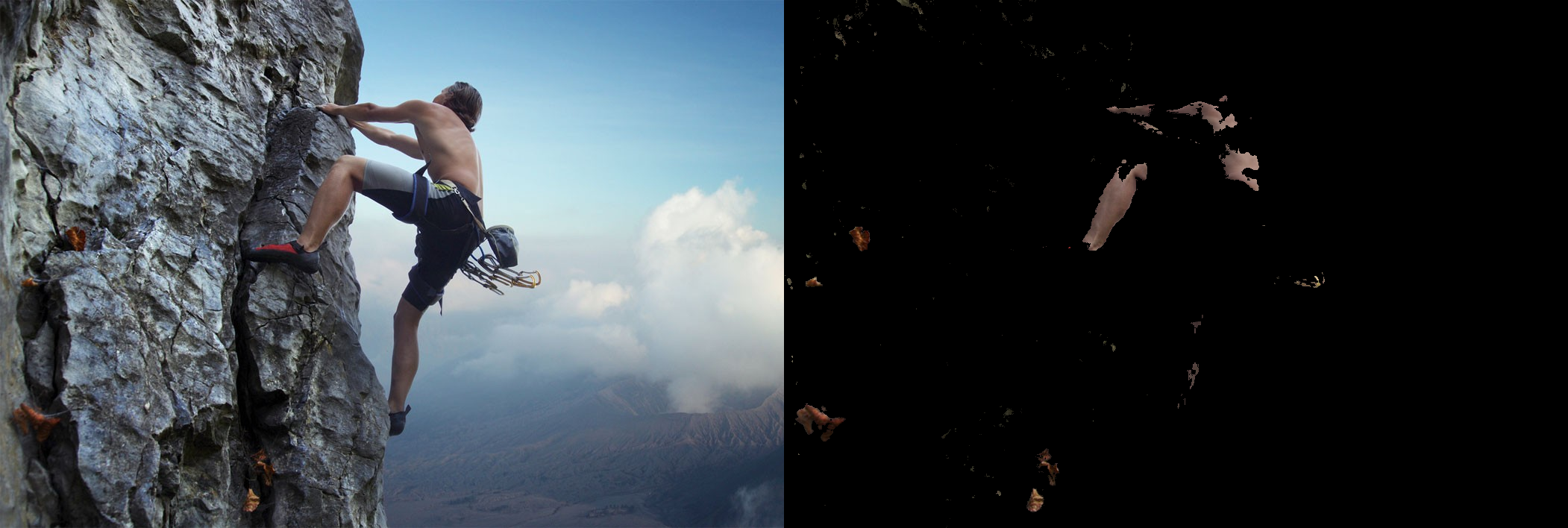# YCrCb Color Space

• Encoded nonlinear RGB signal, commonly used in video coding and image compression work.
• constructed as a weighted sum of the RGB values, and two color difference values Cr and Cb that are formed by subtracting luma from RGB red and blue components
• 16 to 235 for Y, 16 to 240 for Cb and Cr
• Skin pixels form a compact cluster in the Cb-Cr plane.

Transformation simplicity and explicit separation of luminance and chrominance components makes this colorspace attractive for skin color modelling

# Required modules
import cv2
import numpy as np
import matplotlib.pyplot as plt

min_YCrCb = np.array([0,133,77],np.uint8)
max_YCrCb = np.array([235,173,127],np.uint8)

# Get pointer to video frames from primary device
imageYCrCb = cv2.cvtColor(image,cv2.COLOR_BGR2YCR_CB)
skinRegionYCrCb = cv2.inRange(imageYCrCb,min_YCrCb,max_YCrCb)

skinYCrCb = cv2.bitwise_and(image, image, mask = skinRegionYCrCb)

cv2.imwrite("/assets/img/skin-detection/ycrcb.png", np.hstack([image,skinYCrCb]))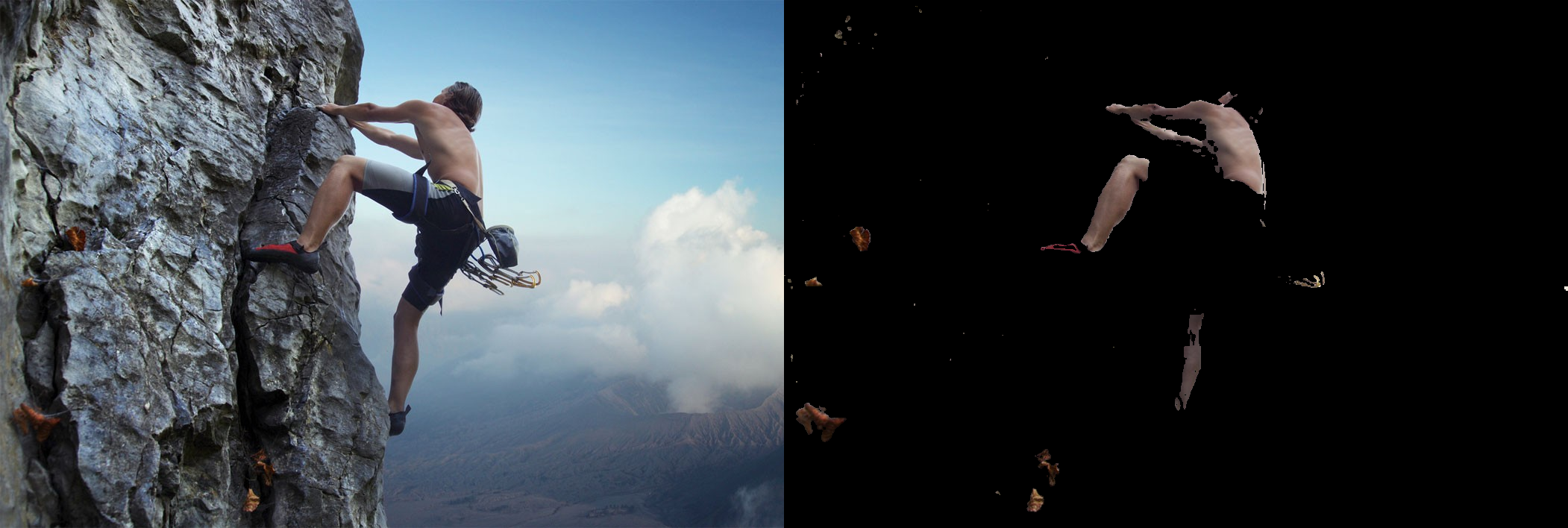# Histogram Calculation

• OpenCV
• cv2.calcHist(images, channels, mask, histSize, ranges)
• images : surce image of type uint8 or float32.
• channels : Index of channel for which we calculate histogram.
• mask : mask image. To find histogram of full image, it is given as “None”.
• histSize : this represents our BIN count. For full scale, we pass .
• ranges : this is our RANGE. Normally, it is [0,256].
• Numpy
• hist,bins = np.histogram(img.ravel(),256,[0,256])
**OpenCV function is faster than (around 40X) than np.histogram(). So stick with OpenCV function.**
# HSV Color-Space Histogram
color = ('r','g','b')
for i,col in enumerate(color):
histr = cv2.calcHist([skinHSV],[i],None,,[0,256])
plt.plot(histr,color = col)
plt.xlim([0,256])
plt.ylim([0,500])
plt.show()# YCrCb Color-Space Histogram
color = ('r','g','b')
for i,col in enumerate(color):
histr = cv2.calcHist([skinYCrCb],[i],None,,[0,256])
plt.plot(histr,color = col)
plt.xlim([0,256])
plt.ylim([0,500])
plt.show()# Histogram Backprojection

• Original algorithm was proposed by Swain and Ballard in their article “Color Indexing” in 1991.
• Backprojection answers to the question “Where in the image are the colors that belong to the object being looked for (the target)?”
• Given the image histogram I and the model histogram M, we define a ratio histogram R as: $R_i = min(\frac{M_i}{I_i},1)$
• function h(c) maps color c of the pixel at (x,y) to the value of the histogram R it indices.
• In other words, function h(c) backprojects the histogram R onto the input image.
• The backprojected image B is then convolved with a disk D of radius r.

#### But how can it be really useful ?

• We can calculate the histogram of an area on a frame, then the same area on another frame from another point of view is more likely to have the same histogram.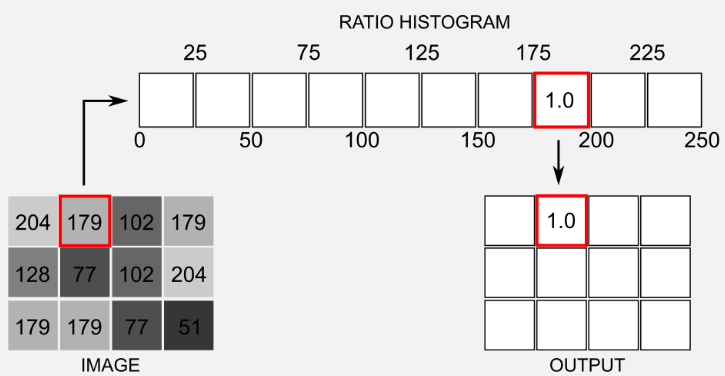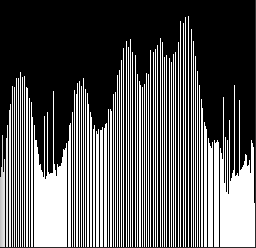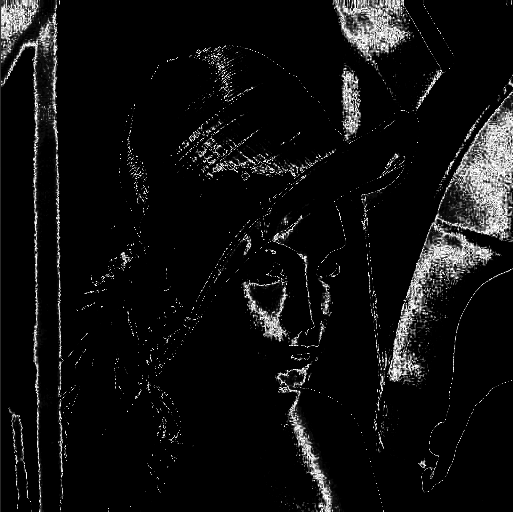def convolve(B, r):
D = cv2.getStructuringElement(cv2.MORPH_ELLIPSE,(r,r))
cv2.filter2D(B, -1, D, B)
return B

image_hsv = cv2.cvtColor(image,cv2.COLOR_BGR2HSV)
model_hsv = image_hsv[225:275,625:675] # Select ROI

#Get the model histogram M
M = cv2.calcHist([model_hsv], channels=[0, 1], mask=None,
histSize=[80, 256], ranges=[0, 180, 0, 256] )

#Backprojection of our original image using the model histogram M
B = cv2.calcBackProject([image_hsv], channels=[0,1], hist=M,
ranges=[0,180,0,256], scale=1)

B = convolve(B, r=5)

#Threshold to clean the image and merging to three-channels
_, thresh = cv2.threshold(B, 30, 255, cv2.THRESH_BINARY)
cv2.imwrite("/assets/img/skin-detection/roi.png",cv2.cvtColor(model_hsv,cv2.COLOR_HSV2RGB))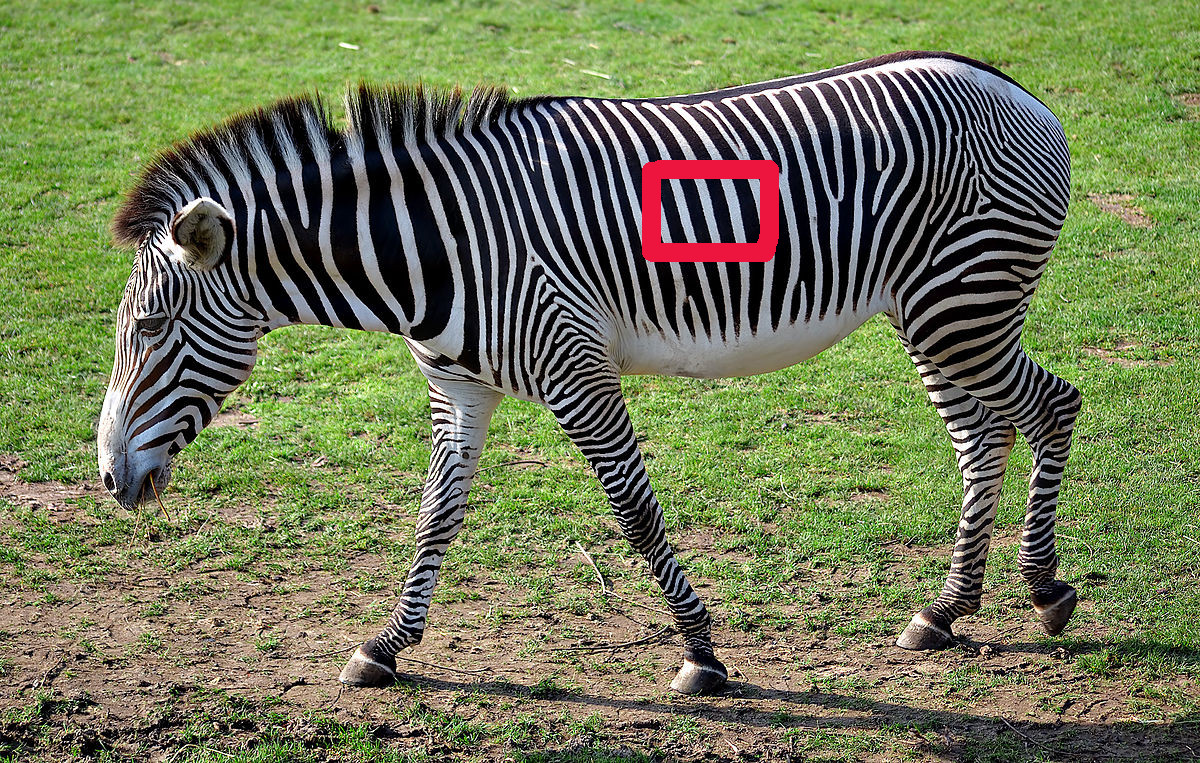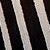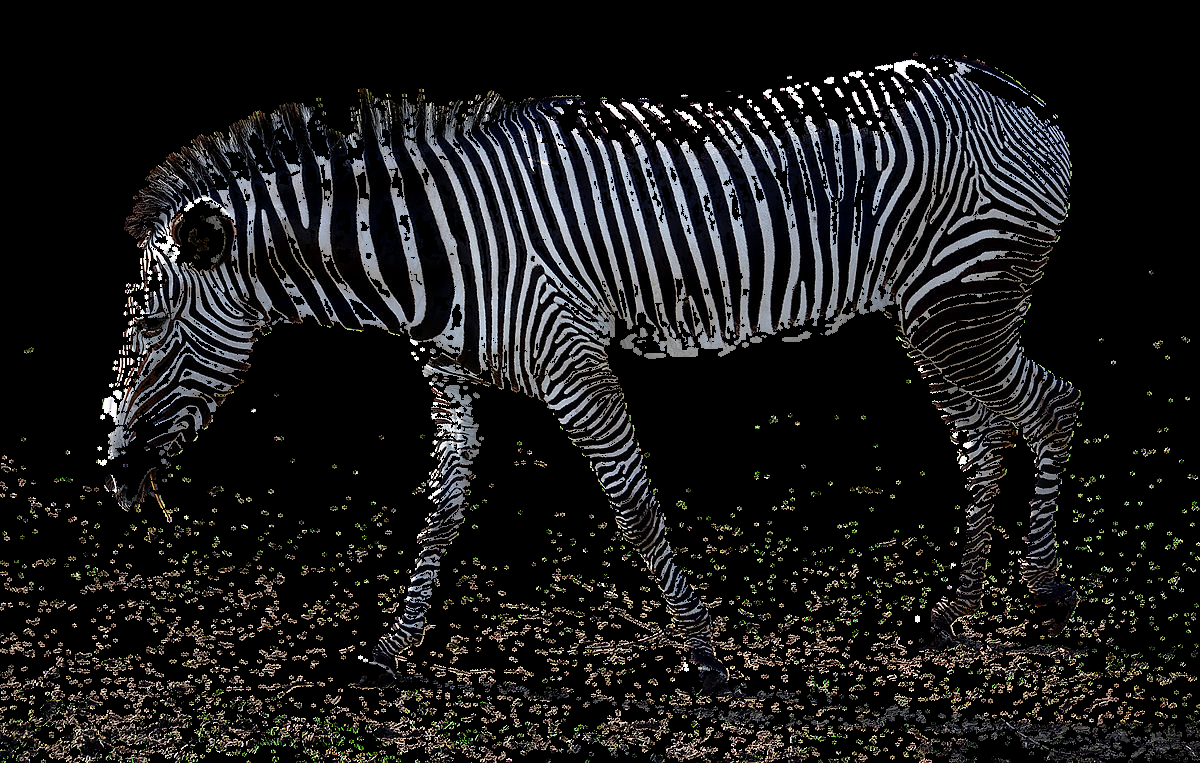image2 = cv2.imread('/assets/img/skin-detection/zebra2.jpg')
image_hsv2 = cv2.cvtColor(image2,cv2.COLOR_BGR2HSV)

#Backprojection on new image using the same model histogram M
B = cv2.calcBackProject([image_hsv2], channels=[0,1], hist=M,
ranges=[0,180,0,256], scale=1)

B = convolve(B, r=5)

#Threshold to clean the image and merging to three-channels
_, thresh = cv2.threshold(B, 30, 255, cv2.THRESH_BINARY)

#Using B_tresh as a mask for a binary AND with the original image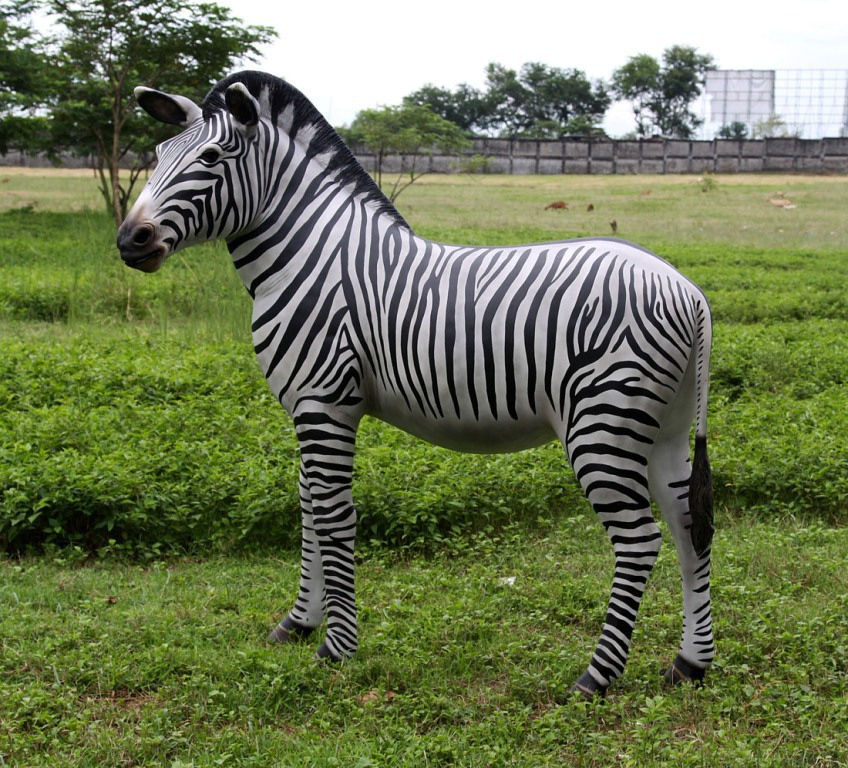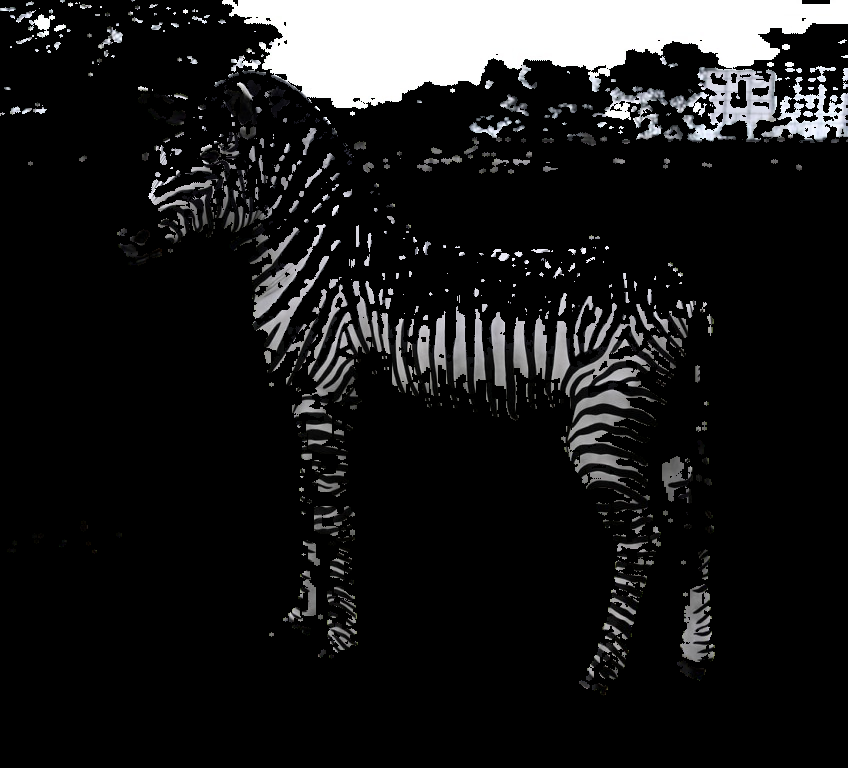## OpenCV

• ### Constructor and Destructor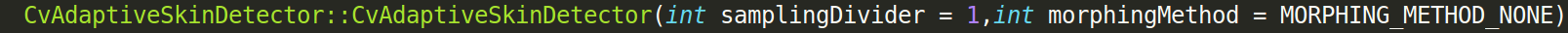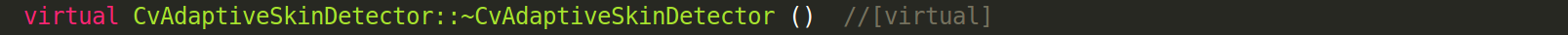• ### Member Functions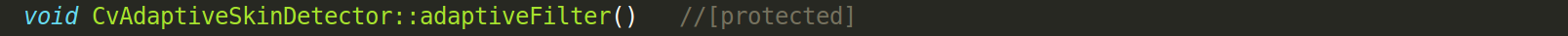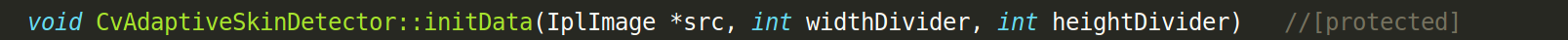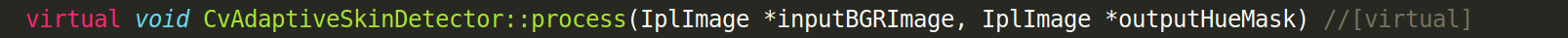Not available in OpenCV 3.x.x

# Why was CvAdaptiveSkinDetector removed from OpenCV 3.x.x ?

### IRC #opencv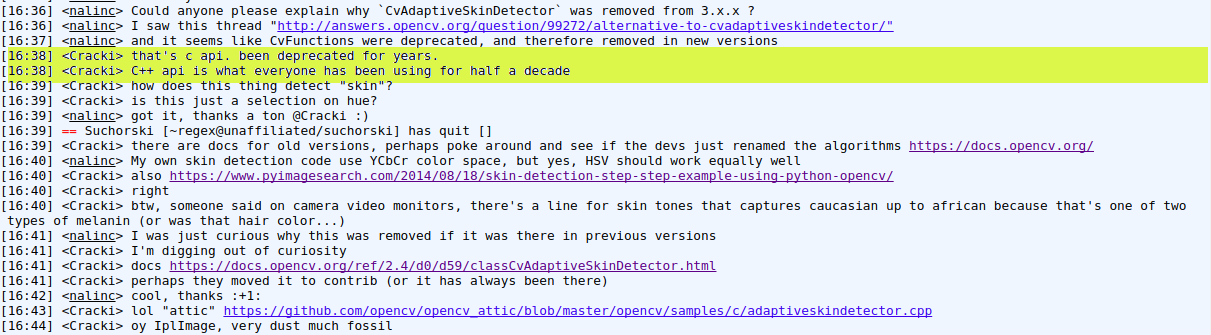# Pros

• Range of colors based skin detection is invariant to orientation and size and is fast to process.
• Useful as a pre-processing step

# Cons

• Most of the techniques report about 95% true detection.
• False detection rates varies from 15 to 30%
• Not useful with gray-scale, infrared, or other types of image modalities that do not contain color information. (Regardless of the choice of the color space and the classification method)

# Applications

### Gesture tracking, Life saving, Pokedex, Games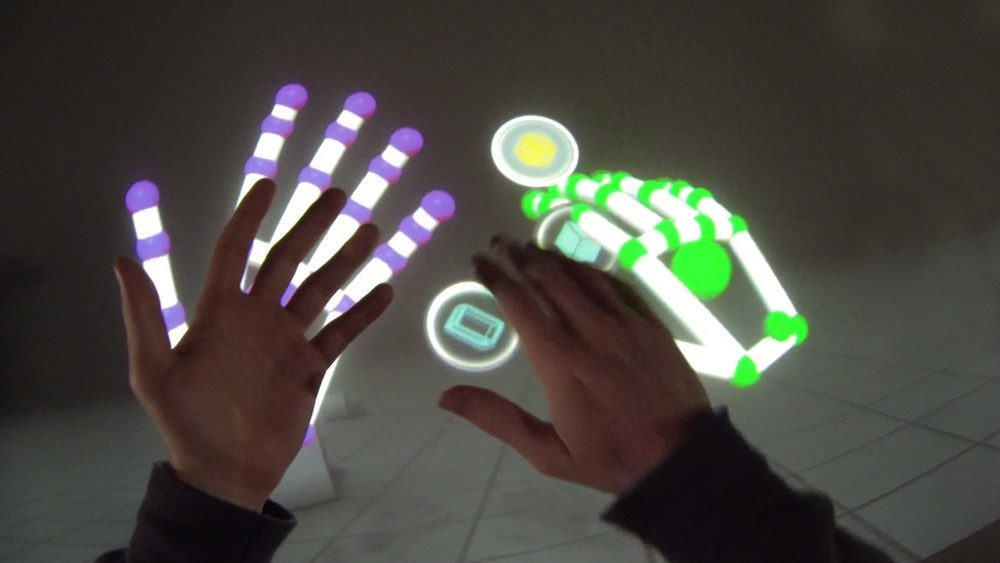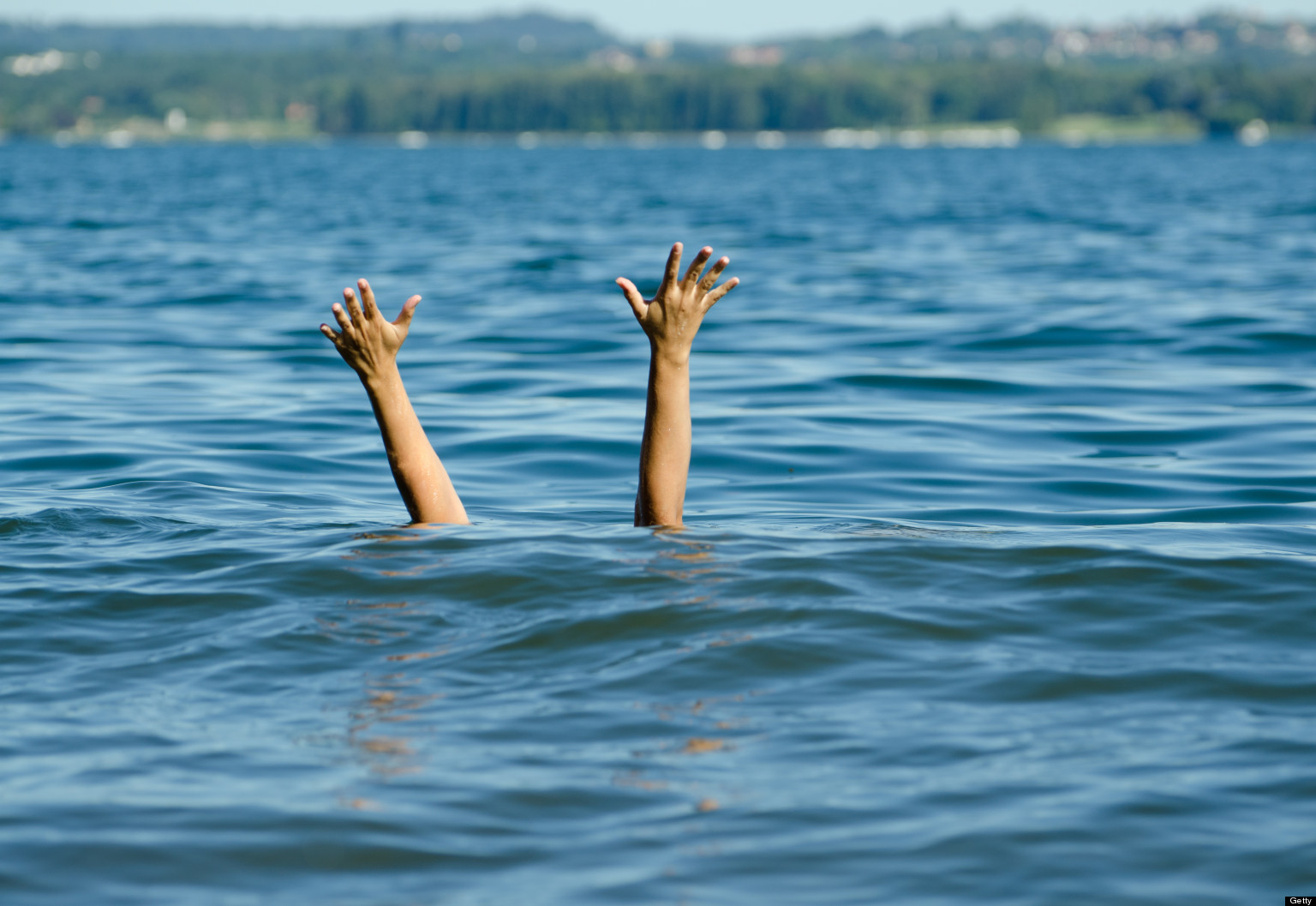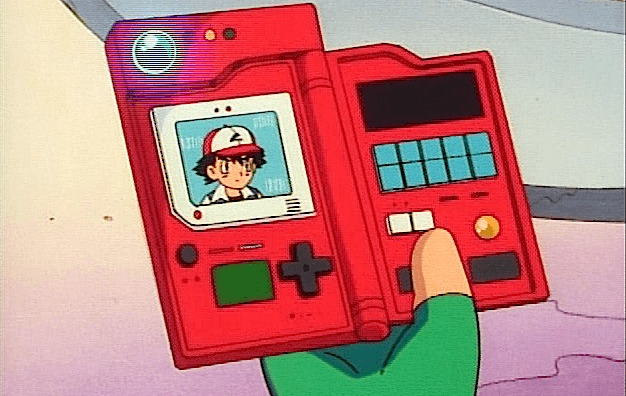# Human skin as a game controller

# Required moduls
import cv2
import numpy
from pykeyboard import PyKeyboard

# Constants for finding range of skin color in YCrCb
min_YCrCb = numpy.array([0,133,77],numpy.uint8)
max_YCrCb = numpy.array([255,173,127],numpy.uint8)

font = cv2.FONT_HERSHEY_SIMPLEX
gameflag = False
keyboard = PyKeyboard()

# Create a window to display the camera feed
cv2.namedWindow('Camera Output')

# Get pointer to video frames from primary device
videoFrame = cv2.VideoCapture(0)

while True: # any key pressed has a value >= 0

# Grab video frame, decode it and return next video frame
sourceImage = cv2.flip(sourceImage, 1)

# Convert image to YCrCb
imageYCrCb = cv2.cvtColor(sourceImage,cv2.COLOR_BGR2YCR_CB)

# Find region with skin tone in YCrCb image
skinRegion = cv2.inRange(imageYCrCb,min_YCrCb,max_YCrCb)

skin = cv2.bitwise_and(sourceImage, sourceImage, mask = skinRegion)

# Do contour detection on skin region
im2, contours, hierarchy = cv2.findContours(skinRegion, cv2.RETR_EXTERNAL, cv2.CHAIN_APPROX_SIMPLE)
cv2.rectangle(skin,(0,300),(640,200),(0,0,255),3)
if len(contours) != 0:
contour = max(contours, key = cv2.contourArea)
M = cv2.moments(contour)
if(M["m00"] != 0):
cx = int(M["m10"]/M["m00"])
cy = int(M["m01"]/M["m00"])
area = M["m00"]
if cy < 200:
cv2.circle(skin, (cx, cy), 7, (0, 255, 0), -1)
if gameflag:
keyboard.press_key(' ')
keyboard.release_key(' ')
elif cy > 300:
cv2.circle(skin, (cx, cy), 7, (255, 0, 0), -1)
if gameflag:
keyboard.press_key('Down')
keyboard.release_key('Down')
else:
cv2.circle(skin, (cx, cy), 7, (255, 255, 255), -1)
gameflag = True

# Display the source image
cv2.imshow('Camera Output',skin)

# Check for user input to close program
if cv2.waitKey(5) == 27: # wait 5 millisecond in each iteration of while loop
break

# Close window and camera after exiting the while loop
cv2.destroyWindow('Camera Output')
videoFrame.release()


Here’s a modified version of the classic t-rex game from chrome offline err page. I used my own skin as a game controller.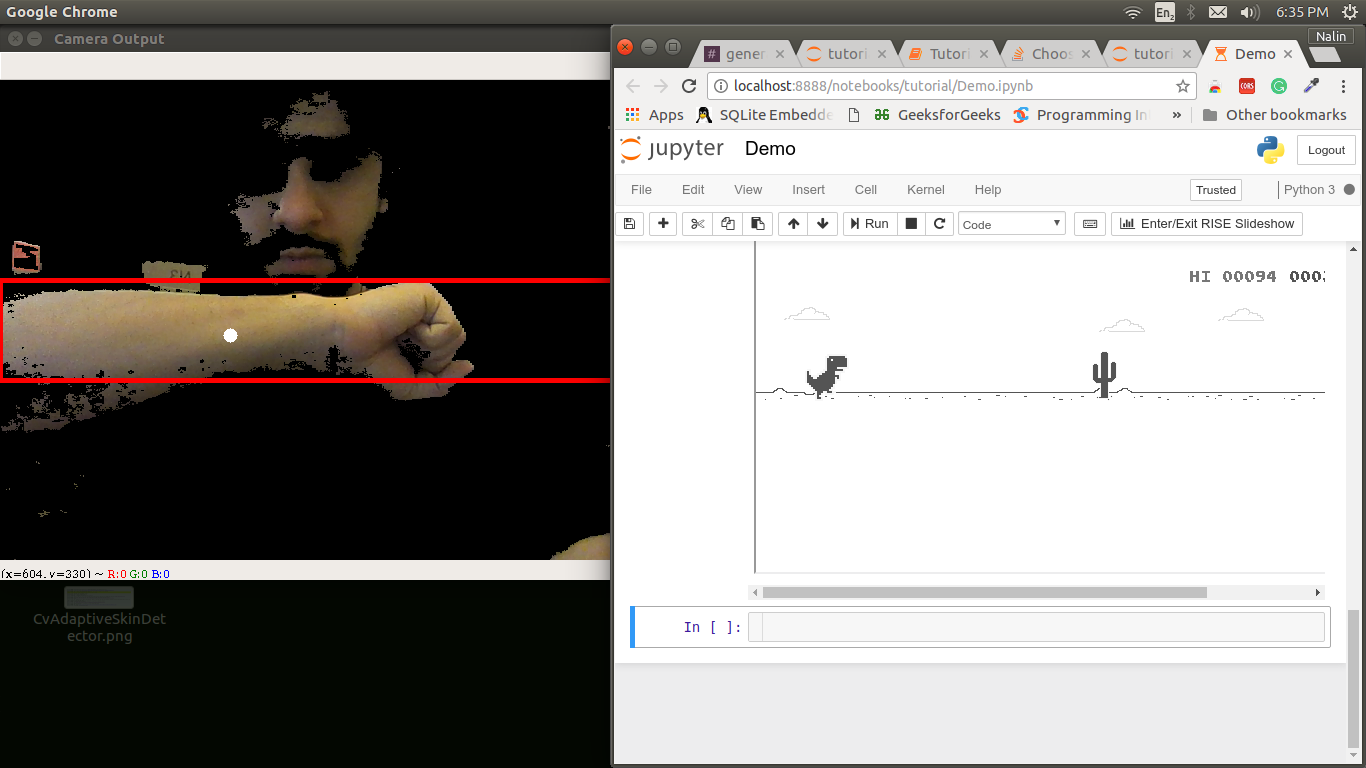Watch the demo below.